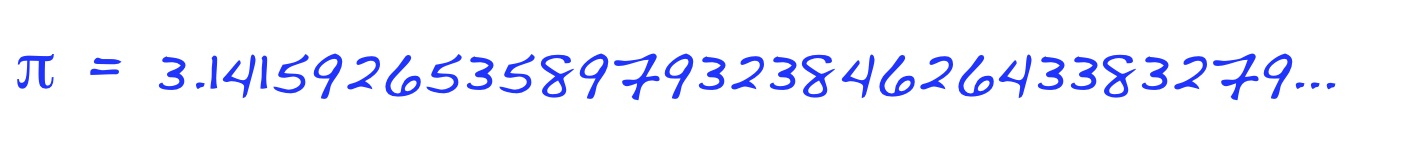# Ï€ does go on forever (unpredictably)

Posted by: Gary Ernest Davis on: January 26, 2011Reuters new agency reported on January 20, here 2011 that a Japanese systems engineer, Shigeru Kondo, had used a home-built computer to calculate$pi$ toÂ  trillions of digits and therebyÂ  broken the existing Guinness Book of Records entry for calculating$pi$.

A sentence toward the end of the report caught my eye:

“Calculating a more accurate pi, which is believed to go on forever, has been a challenge for scholars for thousands of years, ever since the parameter was used in ancient Egypt.” (My emphasis)

####$pi$ is not a rational number

Of course the decimal expansion of$pi$ goes on forever, because$pi$ is not a rational number. That is, there are no integers$m,n$ for which$pi=frac{m}{n}$.

This was first proved by Johann Heinrich Lambert in 1761.

The irrationality of$pi$ means not only does the decimal expansion of$pi$ go on forever, but that it never repeats from some point on.

Of course we are familiar with rational numbers whose decimal expansions repeat, such as$frac{1}{3}=0.333333ldots$,$frac{1}{7}=0.142857142857142857ldots$ and$frac{611}{4950}=0.12343434ldots$ which repeats after the first two decimal places.

Equally, any decimal that eventually repeats is a rational number.

The reason for this is fairly straightforward.

First, if$x$ is a number that has a repeating decimal after, say, the$n^{th}$ decimal place then$10^n imes x$ is a repeating decimal.

Second, repeating decimals$y$ are rational numbers – just multiply$y$by$10^p$ where$p$ is the length of the repeating block, to get$10^p imes y= extrm{ [repeating block] } + y$.

For example, if$x = 0.21356565656ldots$ then$1000 imes x = 213+0.565656ldots$ and if$y= 0.565656ldots$ then$100y=56.565656ldots = 56+y$ so$99 imes y = 56$. So$y$ is a rational number, and so therefore is$x$.

All this means that not only does the decimal expansion of$pi$ go on forever, it never eventually repeats.

####$pi$ is transcendental

The same is true, of course, for$sqrt{2}$ which is an irrational number.

What distinguishes$pi$ from a number such as$sqrt{2}$ is that the latter is algebraic.

A number$heta$ is “algebraic” if there is a polynomial$p(x)=a_0+a_1x+a_2x^2+ldots+a_nx^n$

all of whose coefficients$a_i$ are integers, for which$p( heta )=0$

Of course, in the case of$sqrt{2}$ such a polynomial is$p(x)=x^2-2$.

For$pi$ there is no such polynomial. This is what is means for$pi$ to be a transcendental number:$pi$ is not algebraic.

This was first proved by Ferdinand von Lindemann in 1882.

Algebraic numbers are in many respects easier to understand than non-algebraic (= transcendental ) numbers. In fact, Leopold Kronecker denied the existence of transcendental numbers (including$pi$).

#### Are the digits of$pi$ random?

This is a very difficult question to answer unless we have a workable definition of “random”.

Gregory Chaitin and Andrey Nikolaevich Kolmogorov came up with a definition of “algorithmic randomness” which has to do with the non-compressibility of the description of a number. While this is an interesting and useful idea it does not seem to correspond exactly to what most people think of as randomness.

In practice, a sequence of numbers – such as the digits of$pi$ – is NOT random if it fails one of a batch of tests for randomness.

What this means in practice is that we cannot prove that a sequence of numbers is random, only that it is not random.

If a sequence of numbers passes all known tests for randomness we tentatively conclude that the sequence is behaving like a random sequence.

A 2001 research report by Paul Preuss at Berkley, indicates that the sequence of digits of$pi$ does behave as if it were random.

So not only, do the digits of$pi$ go on forever, they do not eventually repeat, and appear to all intents and purposes, to be random.

### 6 Responses to "Ï€ does go on forever (unpredictably)"[…] This post was mentioned on Twitter by Tito Eliatron, MiGUi and Juan Malagon, Republic of Math. Republic of Math said: Ï€ does go on forever (unpredictably) #pi #math http://wp.me/pJESd-18b […]I enjoy asking my students this question –

True or False: since the digits of pi ‘go on forever’, pi has no definite location on the number line.

Many students consider that a true statement. “How can pi have a definite location if it ‘never ends’?” they ask. I ask them, “So you think it creates a smudge on the number line?” They’re not sure. It’s a very interesting discussion to have, and I think very important. I see how they can form an idea like that from how we use our terms. I try to make it clear to them in the course of discussion that pi does in fact have a specific value and a specific location on the number line – it is the decimal REPRESENTATION that ‘goes on forever’.

– MichelMichel,

I did wonder about writing on this. You are absolutely right.

A question is what people think$\pi$ is: is it the decimal representation (if so, we only know so much about it, and may never know everything); is it the area of a unit circle? If so, what tells us a unit circle has an area? We can of course use inner and outer measures and limits to show that a circle HAS an area, and that area is called$\pi$, but that’s a bit sophisticated for beginners.

There are many other ways to approach$\pi$. For example, as the first positive number$x$ for which$\sin (x)=0$.

Whatever approach we use,$\pi$ comes out as a real number.

Of course, Kronecker did not agree.If x = 0.2135656565656…, then 1000 * x = 213 + 0.56565656…Thanks! Fixed.An informative reading on interesting facts about$\pi$. We will indeed try to estimate its value later in SYE 6005.